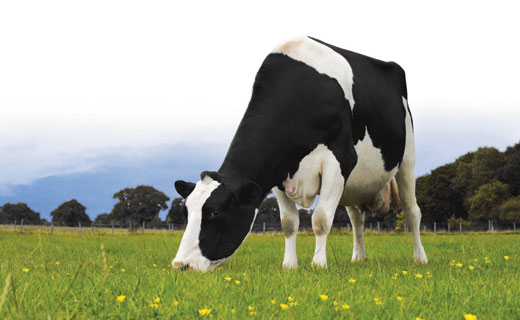# Grazing Cow

Geometry Level 4A cow is tied to a corner (vertex) of a regular hexagonal fenced area of sides $4$ meters long, with a rope of length $10$ meters long, in a grass field. The cow cannot graze inside the fenced area. What is the maximum possible area of grass field in which the cow can graze?

Let $A$ be the area in square meters. Find $\left\lfloor A \right\rfloor$. where $\left\lfloor ... \right\rfloor$ represents Greatest Integer Function.

###### This is an old KVPY problem. Try more such problems here.
×

Problem Loading...

Note Loading...

Set Loading...